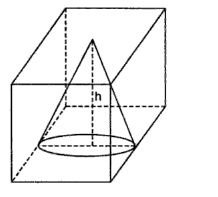Courses
Courses for Kids
Free study material
Free LIVE classes
MoreLIVE
Join Vedantu’s FREE Mastercalss

# Find the volume of the largest right circular cone that can be cut out of a cube whose edge is 9 cm.Verified
360.6k+ views
Hint: The largest circular cone that can be cut out of the cube will have height equal to cube edge and base diameter of cone will be equal to edge of the cube.

Largest right circular cone will have the same height. Let this height be h.
Diameter of the base of the cone is equal to the edge of the cube since it touches all the edges of the cube side.
$\Rightarrow h = 9cm\;\& \;r = \dfrac{{diameter}}{2} = \dfrac{9}{2} = 4.5cm$
Volume $= \dfrac{1}{3}\pi {r^2}h$
$\Rightarrow Volume = \dfrac{1}{3}\pi {\left( {4.5} \right)^2}9 = 190.85c{m^3}$
$\therefore$ The volume of the largest right circular cone formed from the given cube is $190.85c{m^3}$